Geometry

# Regular Polygons - Decomposition into Triangles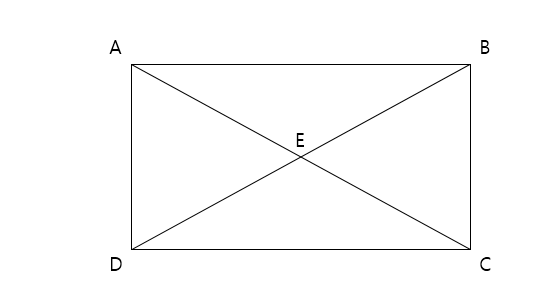Consider a rectangle with diagonals as shown in the above diagram. If the area of $\triangle BCE$ is $32,$ what is the area of $\triangle ABE?$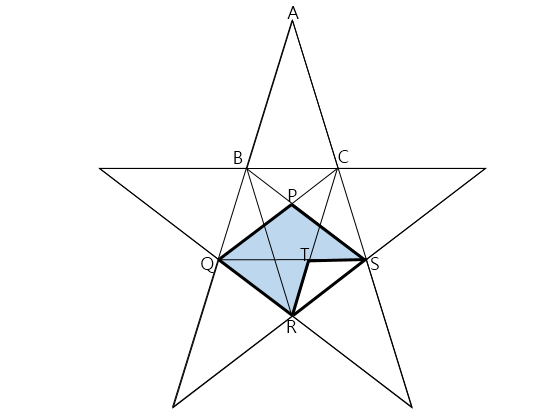The above diagram is a regular pentagram. Let $\lvert\triangle TRS\rvert$ denote the area of $\triangle TRS,$ and $\lvert\square PQRS\rvert$ the area of $\square PQRS.$ If $\lvert\triangle ABC\rvert=52,$ what is the area of the shaded region $\lvert\square PQRS\rvert - \lvert\triangle TRS\rvert?$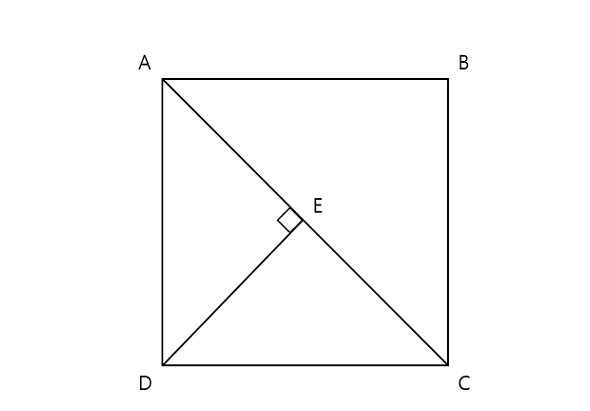In the above diagram, $\square ABCD$ is a square and $\overline{DE}$ is perpendicular to one of its diagonals $\overline{AC}.$ If the length of $\overline{DE}$ is $21.$ what is the length of $\overline{AC}?$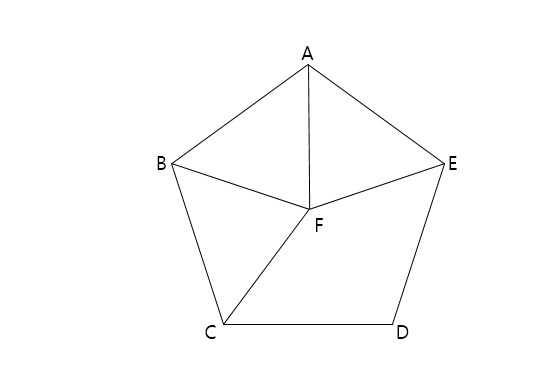In the above diagram, figure $ABCDE$ is a regular pentagon centered at point $F$. Find the area of quadrilateral $CDEF$ when the area of triangle $ABF$ is $56.$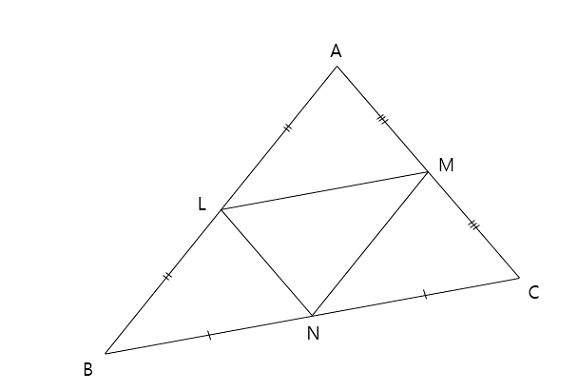In $\triangle ABC$ above, $L,M$ and $N$ are the midpoints of $\overline {AB}, \overline {BC}$ and $\overline {AC},$ respectively. If the area of $\triangle LMN$ is $54,$ what is the area of $\triangle ABC?$

×